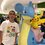# Formula for Root Product

In solving this problem, I found a formula for a product $(1+x_{1})(1+x_{2})(1+x_{3})(1+x_{4})...(1+x_{d})$ up until the last root of a polynomial of degree d. I challenge Brilliant members to find this formula, with proof.Note by Tristan Shin
7 years ago

This discussion board is a place to discuss our Daily Challenges and the math and science related to those challenges. Explanations are more than just a solution — they should explain the steps and thinking strategies that you used to obtain the solution. Comments should further the discussion of math and science.

When posting on Brilliant:

• Use the emojis to react to an explanation, whether you're congratulating a job well done , or just really confused .
• Ask specific questions about the challenge or the steps in somebody's explanation. Well-posed questions can add a lot to the discussion, but posting "I don't understand!" doesn't help anyone.
• Try to contribute something new to the discussion, whether it is an extension, generalization or other idea related to the challenge.
• Stay on topic — we're all here to learn more about math and science, not to hear about your favorite get-rich-quick scheme or current world events.

MarkdownAppears as
*italics* or _italics_ italics
**bold** or __bold__ bold
- bulleted- list
• bulleted
• list
1. numbered2. list
1. numbered
2. list
Note: you must add a full line of space before and after lists for them to show up correctly
paragraph 1paragraph 2

paragraph 1

paragraph 2

[example link](https://brilliant.org)example link
> This is a quote
This is a quote
    # I indented these lines
# 4 spaces, and now they show
# up as a code block.

print "hello world"
# I indented these lines
# 4 spaces, and now they show
# up as a code block.

print "hello world"
MathAppears as
Remember to wrap math in $$ ... $$ or $ ... $ to ensure proper formatting.
2 \times 3 $2 \times 3$
2^{34} $2^{34}$
a_{i-1} $a_{i-1}$
\frac{2}{3} $\frac{2}{3}$
\sqrt{2} $\sqrt{2}$
\sum_{i=1}^3 $\sum_{i=1}^3$
\sin \theta $\sin \theta$
\boxed{123} $\boxed{123}$

## Comments

Sort by:

Top Newest

Let the polynomial be $f(x)$ with degree $n$ and roots $r_1, r_2, \dots, r_{n-1}, r_{n}$

Suppose $f(x) = a_0x^n + a_1x^{n-1} + \dots + a_{n-1}x + a_n = a_0(x-r_1)(x-r_2)\dots(x-r_{n-1})(x-r_{n})$

Then $f(-1) = a_0(-1)^n + a_1(-1)^{n-1} + \dots + a_{n-1}(-1) + a_n = a_0(-1-r_1)(-1-r_2)\dots(-1-r_{n-1})(-1-r_n)$

$f(-1) = a_0(-1)(1+r_1)(-1)(1+r_2)\dots(-1)(1+r_{n-1})(-1)(1+r_n)$

$\dfrac{f(-1)}{a_0} = (-1)^n(1+ r_1)(1 + r_2)\dots(1+ r_{n-1})(1+ r_n)$

$(1+ r_1)(1 + r_2)\dots(1+ r_{n-1})(1+ r_n) = \left\{ \begin{array}{lr} \frac{-f(-1)}{a_0} & : n \equiv 1 \pmod2 \\ \frac{f(-1)}{a_0} & : n \equiv 0 \pmod2 \end{array} \right.$

Log in to reply

The solution is almost complete. The third time you state $f\left(-1\right)$, this can be simplified after in the next step. In addition, what if there is a coefficient of the $x^{n}$ term? Consider these and make an edit.

- 7 years ago

Log in to reply

I don't understand what you meant in the first line. I've edited for the case when there is a coefficient of the leading term.

Log in to reply

Your solution is just one thing away. Instead of using modular arithmetic in your final formula, what can you do with the sign instead?

- 7 years ago

Log in to reply

Are you implying $(-1)^n$?

- 7 years ago

Log in to reply

I'll let some more people try, then I'll post this answer sometime next week(April 14 to 18).

- 7 years ago

Log in to reply

It seems that Siddhartha Srivastava has come very close to solving this problem. Congratulations!

- 7 years ago

Log in to reply

There is a pattern.....for example...............

( a + 1 ) ( b + 1 ) ( C + 1 ) = 1 + a + b + c + a b + b c + c a + a b c .

Hope you want this!

- 7 years ago

Log in to reply

Yes, this is the pattern, but it can be simplified even further. Think about the certain terms in your example.

- 7 years ago

Log in to reply

Well, Tristan, I can't get it......please post the answer.....

- 7 years ago

Log in to reply

it would be kind enough if u tell us......

- 7 years ago

Log in to reply

×

Problem Loading...

Note Loading...

Set Loading...